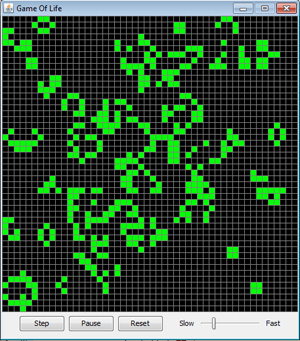## Game of life

 Eine Population entwickelt sich nach folgenden Regeln: Jede Zelle hat 8 Nachbarzellen. jede lebende Zelle, die keinen oder nur einen Nachbarn hat, stirbt (einsam) jede lebende Zelle, die 4 oder mehr Nachbarn hat, stirbt (überbevölkert) jede lebende Zelle, die 2 oder 3 Nachbarn hat überlebt eine tote Zelle, die 3 Nachbarn hat, wird lebendig Implementierung: Zu Beginn werden zufällig 1000 lebende Zellen bestimmt. Diese sind grün gefärbt. In jedem Simulationstzyklus wird für jede Zelle die Anzahl der Nachbaren bestimmt und die Zelle als lebend (grün) oder als Tod (schwarz) markiert.```# Game of Life from gamegrid import * class Game(GameGrid): def __init__(self): GameGrid.__init__(self, s, s, 800 // s, Color.gray) self.setTitle("Game Of Life") self.reset() self.show() def reset(self): # Called when the reset button is clicked for x in range(s): for y in range(s): a[x][y] = 0 # All cells dead for n in range(z): loc = self.getRandomEmptyLocation() a[loc.x][loc.y] = 1 self.showPopulation() def showPopulation(self): for x in range(s): for y in range(s): loc = Location(x, y) if a[x][y] == 1: self.getBg().fillCell(loc, Color.green, False) else: self.getBg().fillCell(loc, Color.black, False) def getNumberOfNeighbours(self, x, y): nb = 0 for i in range(max(0, x - 1), min(s - 1, x + 2)): for k in range(max(0, y - 1), min(s - 1, y + 2)): if not (i == x and k == y): if a[i][k] == 1: nb = nb + 1 return nb # Automatically called at the end of each simulation cycle def act(self): global a b = [[0 for x in range(s)] for y in range(s)] for x in range(s): for y in range(s): nb = self.getNumberOfNeighbours(x, y) if a[x][y] == 1: # live cell if nb < 2: b[x][y] = 0 elif nb > 3: b[x][y] = 0 else: b[x][y] = 1 else: # dead cell if nb == 3: b[x][y] = 1 else: b[x][y] = 0 a = b self.showPopulation() s = 50 # Number of cells in each direction z = 1000 # Size of population at start a = [[0 for x in range(s)] for y in range(s)] Game() ```
Programmcode markieren (Ctrl+C kopieren, Ctrl+V einfügen)